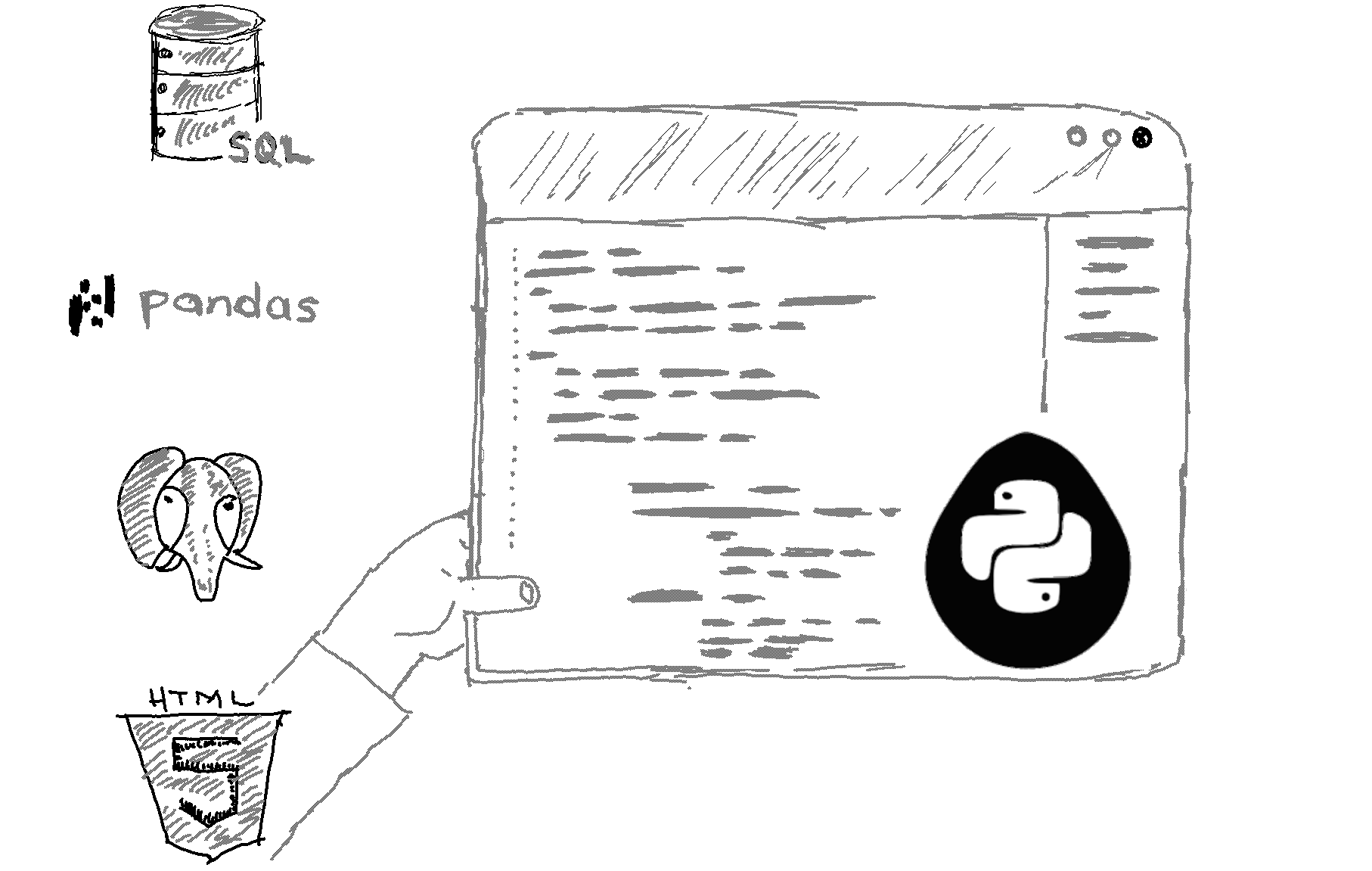# Here is how to convert two lists into a dictionary in Python.

```keys = ['a', 'b', 'c']
values = [1, 2, 3]
my_dict = dict(zip(keys, values))
print(my_dict) ```

Output
`{'a': 1, 'b': 2, 'c': 3}`

Explanation

You can use the zip function to convert two lists into a dictionary. The zip function in Python is used to combine two or more iterables (e.g. lists, tuples, etc.) into a single iterable object.

In this case, we're using zip to combine two lists keys and values into a single iterable. The zip function takes each element of the first iterable, and each corresponding element of the second iterable, and creates a tuple out of them. So, in our example, the first tuple would be ('a', 1).

Finally, we pass the result of the zip function to the dict constructor, which creates a dictionary using the tuples as keys and values. So, in the end, we get a dictionary with the keys 'a', 'b', and 'c' and the values 1, 2, and 3, respectively.

Related HowTos
##### Recommended Course

Learn Flask development and learn to build cool apps with our premium Python course on Udemy.